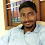# Spark Interview Questions and Answer - Coding Round | Apache Spark Interview

In this blog, we will have some practical working session on the question asked in Spark Interview Question during coding round. This Spark Interview Question is shared with the candidate from the one of the fortune 500 company in India for Data Engineer Role.

Try to answer the question without going through the answers and compare your way of approach to solve this Spark question with the below given approach.

### Question:

Write a script for the below scenario either in PySpark (or) Spark Scala.

#### Note:

• Code only using Spark RDD.
• Dataframe or Dataset should not be used
• Candidate can use Spark of version 2.4 or above

1. Read the input testfile (Pipe delimited) provided as a "Spark RDD"

2. Remove the Header Record from the RDD

3. Calculate Final_Price:

Final_Price = (Size * Price_SQ_FT)

4. Save the final rdd asTextfile with three fields

Property_ID|Location|Final_Price

### Source File:

Input text file is pipe delimited and contains the data related to the real estate of United States. Above shown is the sample of dataset given and required output file and you can be downloaded input text file using below link.

### Solution:

We solve this Spark Interview Question using PySpark as a programming language.

```# Create SparkSession and sparkcontext
from pyspark.sql import SparkSession
spark = SparkSession.builder\
.master("local")\
.appName('Assignment 2')\
.getOrCreate()
sc=spark.sparkContext

#Read the input file as RDD using Spark Context
rdd_in=sc.textFile("input.txt")

#Apply Filter to get header and data
rdd1=rdd_in.filter(lambda l: not l.startswith("Property_ID"))

#Apply Map and flatMap to get the column data
rdd2=rdd1.flatMap(lambda x:x.split(',')).map(lambda x: x.split('|'))

#Get the index of the required column
f1=col_list.index("Property_ID")
f2=col_list.index("Location")
f3=col_list.index("Size")
f4=col_list.index("Price_SQ_FT")

#Function definition to calculate the final price
def mul_price(d1,d2):
res=float(d1)*float(d2)
return str(res)

#Call the function and create final result as expected
rdd3=rdd2.map(lambda x: x[f1]+"|"+x[f2]+"|"+ mul_price(x[f3],x[f4]))

#Save the final Spark RDD as textfile
final_out.coalesce(1).saveAsTextFile("output.txt")

```

### Output Sample:

final_out.collect()

Hope you guys are able to solve this quest without checking for the given solution.

Happy Learnings !!!

1.In 2022 if you are using this approach then you must immediately start learning and upgrading youself in Spark.

1.Hey DEBA's.. Even in 2022, to process the unstructured data we use Spark RDD.. and to stay in bigdata world for long run you should be aware of coding with Spark RDD aswell.

2.Appreciate ur effort Shahul.. Keep going...

3.Hi Shahul.Super Content..Can you please share a scala equivalent for this problem ??